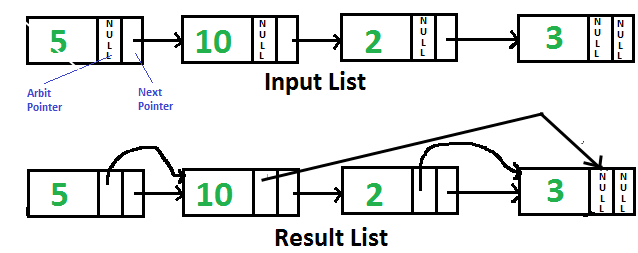GeeksforGeeks App
Open AppBrowser
Continue

# Java Program For Pointing Arbit Pointer To Greatest Value Right Side Node In A Linked List

Given singly linked list with every node having an additional “arbitrary” pointer that currently points to NULL. We need to make the “arbitrary” pointer to the greatest value node in a linked list on its right side.A Simple Solution is to traverse all nodes one by one. For every node, find the node which has the greatest value on the right side and change the next pointer. The Time Complexity of this solution is O(n2).

An Efficient Solution can work in O(n) time. Below are the steps.

1. Reverse the given linked list.
2. Start traversing the linked list and store the maximum value node encountered so far. Make arbit of every node to point to max. If the data in the current node is more than the max node so far, update max.

Following is the implementation of the above steps.

## Java

 `// Java program to point arbit pointers``// to highest value on its right``class` `GfG{` `// Link list node``static` `class` `Node``{``    ``int` `data;``    ``Node next, arbit;``}` `/* Function to reverse the``   ``linked list */``static` `Node reverse(Node head)``{``    ``Node prev = ``null``,``         ``current = head, next = ``null``;``    ``while` `(current != ``null``)``    ``{``        ``next = current.next;``        ``current.next = prev;``        ``prev = current;``        ``current = next;``    ``}``    ``return` `prev;``}` `// This function populates arbit pointer``// in every node to the greatest value``// to its right.``static` `Node populateArbit(Node head)``{``    ``// Reverse given linked list``    ``head = reverse(head);` `    ``// Initialize pointer to maximum``    ``// value node``    ``Node max = head;` `    ``// Traverse the reversed list``    ``Node temp = head.next;``    ``while` `(temp != ``null``)``    ``{``        ``// Connect max through arbit``        ``// pointer``        ``temp.arbit = max;` `        ``// Update max if required``        ``if` `(max.data < temp.data)``            ``max = temp;` `        ``// Move ahead in reversed list``        ``temp = temp.next;``    ``}` `    ``// Reverse modified linked list``    ``// and return head.``    ``return` `reverse(head);``}` `// Utility function to print result``// linked list``static` `void` `printNextArbitPointers(Node node)``{``    ``System.out.println(``"Node    "` `+``                       ``"Next Pointer    "` `+``                       ``"Arbit Pointer"``);``    ``while` `(node != ``null``)``    ``{``        ``System.out.print(node.data +``                         ``"        "``);` `        ``if` `(node.next != ``null``)``            ``System.out.print(node.next.data +``                             ``"        "``);``        ``else``            ``System.out.print(``"NULL"` `+``                             ``"        "``);` `        ``if` `(node.arbit != ``null``)``            ``System.out.print(node.arbit.data);``        ``else``            ``System.out.print(``"NULL"``);` `        ``System.out.println();``        ``node = node.next;``    ``}``}` `/* Function to create a new node``   ``with given data */``static` `Node newNode(``int` `data)``{``    ``Node new_node = ``new` `Node();``    ``new_node.data = data;``    ``new_node.next = ``null``;``    ``return` `new_node;``}` `// Driver code``public` `static` `void` `main(String[] args)``{``    ``Node head = newNode(``5``);``    ``head.next = newNode(``10``);``    ``head.next.next = newNode(``2``);``    ``head.next.next.next = newNode(``3``);` `    ``head = populateArbit(head);` `    ``System.out.println(``           ``"Resultant Linked List is: "``);``    ``printNextArbitPointers(head);``}``}``// This code is contributed by Prerna Saini.`

Output:

```Resultant Linked List is:
Node    Next Pointer    Arbit Pointer
5               10              10
10              2               3
2               3               3
3               NULL            NULL```

Time Complexity: O(n), where n is the number of nodes in the linked list.

Space Complexity: O(1). The algorithm uses a constant amount of extra space to store temporary variables during the traversal of the linked list.

Recursive Solution:
We can recursively reach the last node and traverse the linked list from the end. The recursive solution doesn’t require reversing the linked list. We can also use a stack in place of recursion to temporarily hold nodes. Thanks to Santosh Kumar Mishra for providing this solution.

## Java

 `// Java program to point arbit pointers``// to highest value on its right``class` `GfG``{``    ``// Link list node``    ``static` `class` `Node``    ``{``        ``int` `data;``        ``Node next, arbit;``    ``}` `    ``static` `Node maxNode;` `    ``// This function populates arbit pointer``    ``// in every node to the greatest value``    ``// to its right.``    ``static` `void` `populateArbit(Node head)``    ``{``        ``// if head is null simply return``        ``// the list``        ``if` `(head == ``null``)``            ``return``;` `        ``/* if head->next is null it means we``           ``reached at the last node just update``           ``the max and maxNode */``        ``if` `(head.next == ``null``)``        ``{``            ``maxNode = head;``            ``return``;``        ``}` `        ``/* Calling the populateArbit to the``           ``next node */``        ``populateArbit(head.next);` `        ``/* updating the arbit node of the current``           ``node with the maximum value on the``           ``right side */``        ``head.arbit = maxNode;` `        ``/* if current Node value id greater then``           ``the previous right node then update it */``        ``if` `(head.data > maxNode.data)``            ``maxNode = head;` `        ``return``;``    ``}` `    ``// Utility function to print result``    ``// linked list``    ``static` `void` `printNextArbitPointers(Node node)``    ``{``        ``System.out.println(``"Node    "` `+``                           ``"Next Pointer    "` `+``                           ``"Arbit Pointer"``);``        ``while` `(node != ``null``)``        ``{``            ``System.out.print(node.data +``                             ``"            "``);` `            ``if` `(node.next != ``null``)``                ``System.out.print(node.next.data +``                                 ``"                "``);``            ``else``                ``System.out.print(``"NULL"` `+``                                 ``"            "``);` `            ``if` `(node.arbit != ``null``)``                ``System.out.print(node.arbit.data);``            ``else``                ``System.out.print(``"NULL"``);` `            ``System.out.println();``            ``node = node.next;``        ``}``    ``}` `    ``/* Function to create a new node``       ``with given data */``    ``static` `Node newNode(``int` `data)``    ``{``        ``Node new_node = ``new` `Node();``        ``new_node.data = data;``        ``new_node.next = ``null``;``        ``return` `new_node;``    ``}` `    ``// Driver code``    ``public` `static` `void` `main(String[] args)``    ``{``        ``Node head = newNode(``5``);``        ``head.next = newNode(``10``);``        ``head.next.next = newNode(``2``);``        ``head.next.next.next = newNode(``3``);` `        ``populateArbit(head);` `        ``System.out.println(``               ``"Resultant Linked List is: "``);``        ``printNextArbitPointers(head);``    ``}``}``// This code is contributed by shubham96301   `

Output:

```Resultant Linked List is:
Node    Next Pointer    Arbit Pointer
5               10              10
10              2               3
2               3               3
3               NULL            NULL```

Time complexity: The time complexity of the populateArbit() function is O(n), where n is the number of nodes in the linked list.

Space complexity: The space complexity of the program is O(n), where n is the number of nodes in the linked list.

Please refer complete article on Point arbit pointer to greatest value right side node in a linked list for more details!

My Personal Notes arrow_drop_up